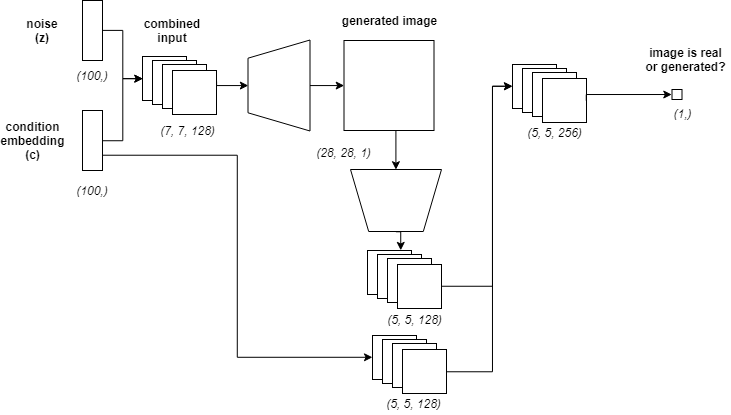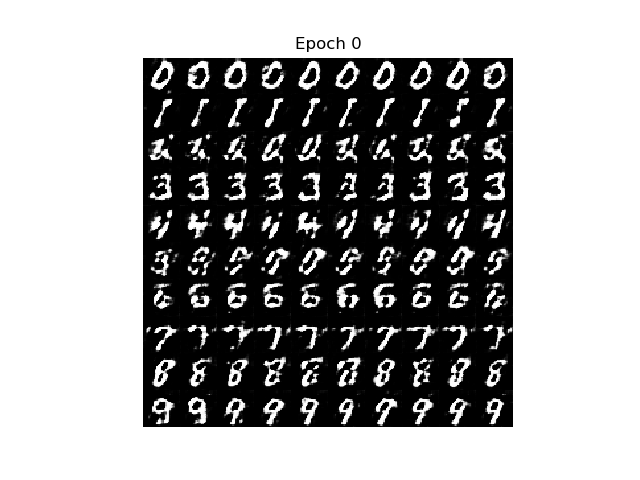# Conditional Deep Convolutional GAN Keras Implementation

## Conditional Deep Convolutional GAN Keras Implementation

- 1 min

In this really short post I will show the overall architecture and the results of a Conditional DCGAN. There are several tutorials and explanations for GANs, so the intention with post is just to showcase the results and share the code.

Implementation can be found here

Why is it conditional?

Because we are using an extra piece of class information besides the random noise input. That means we can control the class of the generated image based on a input label.

## Architecture### Generator $G(z, c)$

The input for the generator is a noise vector $z \in N(0, 1)$ and a condition vector $c$ which describes the label which we want to generate. With $c$ the $G(z, c)$ model will learn the conditional distribution of the data.

### Discriminator $D(X, c)$

The goal of the discriminator is to decide if the input image with the condition vector $c$ came from the original dataset or from $G$.

## Results

Generated digits at every epoch:Linear interpolation results: